# Random Points In A Circle

### When would I ever want to pick a random point in a circle?

Imagine you are working on a First Person Shooter as a Gameplay Engineer. Your job is to implement the shotgun firing mode. That means you need to fire multiple projectiles at once from a single round. Obviously a shotgun would not fire all the projectiles on the same straight line of sight. Shotguns fire rounds that break up into multiple projectiles that are spread out over a certain area. The shape of the area made up by the projectiles is circular because the barrel of a shotgun is circular.

One way we could simulate the shotgun spread mechanic is by allowing a Designer to specify a max radius. We could then pick a random point from the center of our reticle to the max radius.

### How do we get a random point in a circle?

#### The Box Method

There are several ways we could pick a random point in our circle. One way would be to pick a random point in a square. If the distance from the center of the circle to our random point is greater than our Designer’s radius, then we reject it and try again.

###### Code Sample:

Using the box method, we will get results that look uniformly distributed however there is a price to be paid.
There is no way to guarantee how many random points you will end up with unless you sit in a while loop until you have all the points you want. You can use a while loop if you prefer but there is no way to know how long this operation will take. This might be acceptable if you are using the box method during an initialization process but if you need to pick random points in a circle per frame or in an update loop, there are much better ways of doing it.

#### Polar Coordinates

A more efficient way to pick a random point in a circle is to use polar coordinates. Typically, we use what are called Cartesian coordinates to pick a point on a graph. Cartesian coordinates have an x and y component. We move horizontally by however many units are specified by the x value and likewise do the same vertically based on how many units are specified by the y component.

Polar Coordinates are just another way to pick points on a graph.
Instead of moving linearly both horizontally and vertically, Polar Coordinates allow us to move more easily around a circle.  A Polar Coordinate has 2 components similarly to the Cartesian Coordinate system.  Polar Coordinate look like, where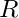is the radius or distance from the center point of the circle and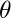is the angle about which we rotate around the center point.

Figure 3.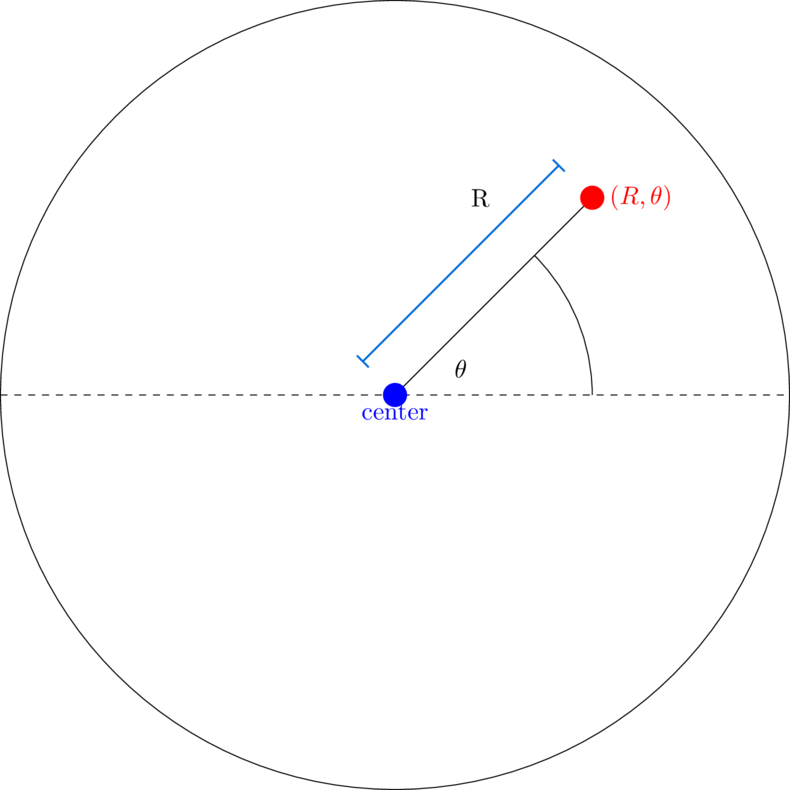To pick a random point using polar coordinates, we just need to pick a random radius and random angle.
We can do so in code like so,

###### Code Sample:

but this will give us an output like this

Notice how we chose more points towards the center of the circle than towards that outer edges of the circle.  For some games, this spread may be appropriate. However what if you wanted your points to be uniformly spread out and not so dense in the center?

So why does randomly choosing an angle and radius make our random point selection more dense towards the center? Imagine you only have 50 points and you want to randomly distribute them about a very small circle. You will probably be able to do that just fine. However as you scale the circle out, you will start to have more and more area that you need to cover but not enough points to cover all the area because you are limited to only 50 points. Take a look at Figure 6 to visualize this.

Figure 6.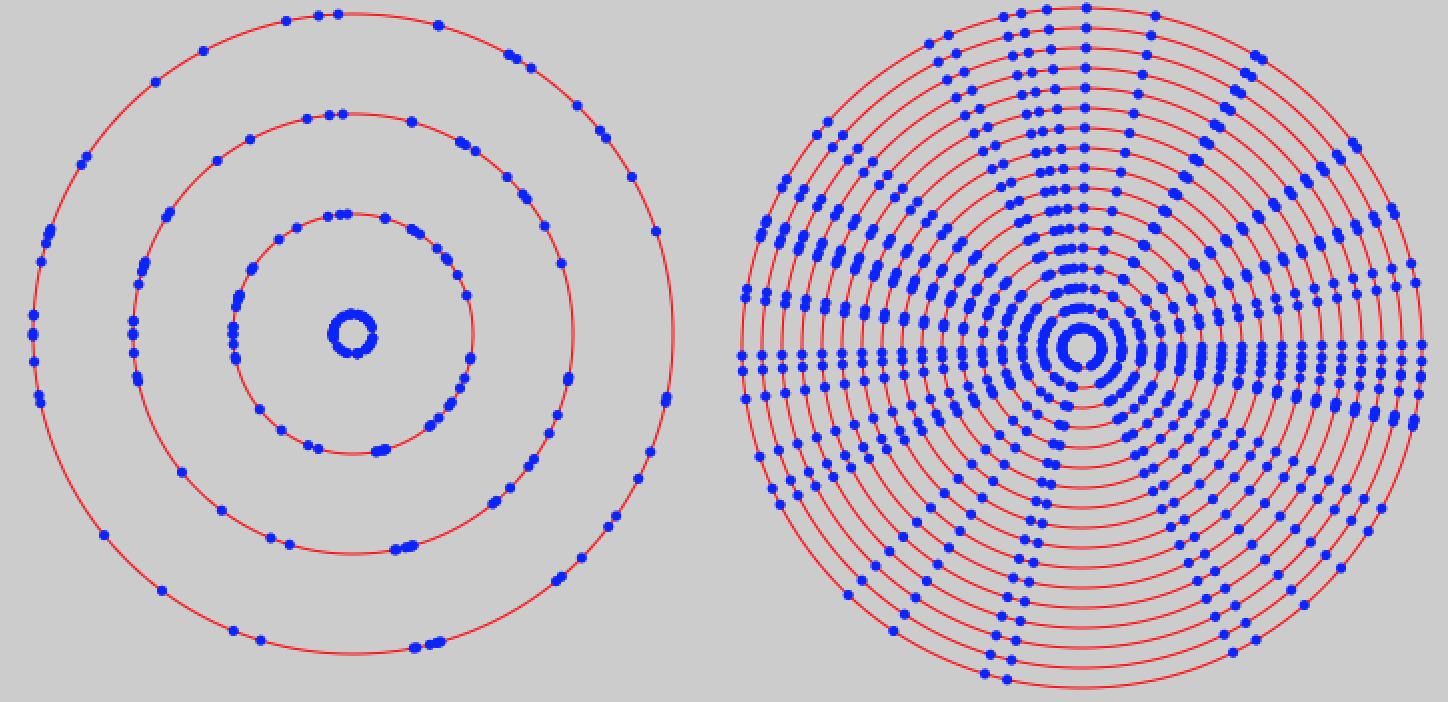### Exploiting the Area of a Circle

We were on the right track when using polar coordinates to pick a randomly distributed set of points in our circle. The only problem that stood in our way was the fact that our random points were more dense toward the center of the circle. The fundamental problem is that we are not uniformly covering all of the circle’s area. The random angle we chose should be fine because an angle has nothing to do with the area of the circle. However the radius cuts through the area of the circle. Therefore we want to focus in on how we can randomly pick a better radius. We need to somehow ensure that the probability of the radius distance is proportional to the area of the circle. In other words, we need to ensure that our random function takes into account the area of our circle.  If only we could multiply our desired radius by some random weight that took our circle’s area into consideration…

Lets start by thinking about what the area of a circle is.
The formula for the area of a circle is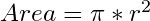.
The area of a circle is agnostic to any kind of probability. So if we could derive the formula for the radius based on the area of a circle, we should be able to randomly distribute our points in a uniform fashion.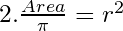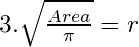Now pay close attention to the relationship between the the fraction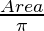and the area of a circle which is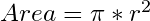.

In both math and philosophy, it is often wise to test the limits or extreme cases of a statement to find out whether that statement is logically consistent and what ultimate conclusions that statement may lead to.

What would be the area of our circle if our radius was 1?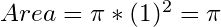.

So forget about what the *actual* radius is of our circle. Lets keep things simple and pretend that our circle’s radius is between 0 and 1. Our fractionmeans that when the Area is 0,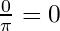and when the area is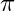, then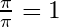. However since we are taking the square root of our fraction, it gives less weight to lower values than does the earlier linear version we tried. That is exactly what we want because the problem we were running into earlier was that we gave too much weight to points closer to the center of the circle and not enough weight towards the edge of the circle. Take a look at Figure 7 to visualize the difference between square root vs linear weighting.

Figure 7.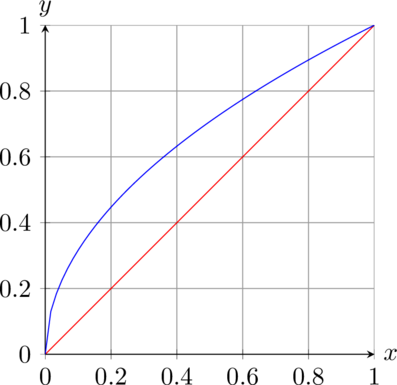So really we can substitute our fraction,, under the square root with any number between the values of 0 to 1.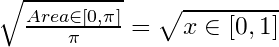As seen in the graph in Figure 7, the value of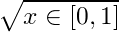will still be within the range of 0 to 1 which we can use as a percentage scalar to multiply by the actual radius!

Therefore to pick a random uniformly distributed point in a circle using polar coordinates, we can do the following,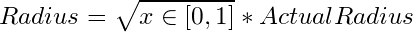Angle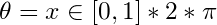Figure 8.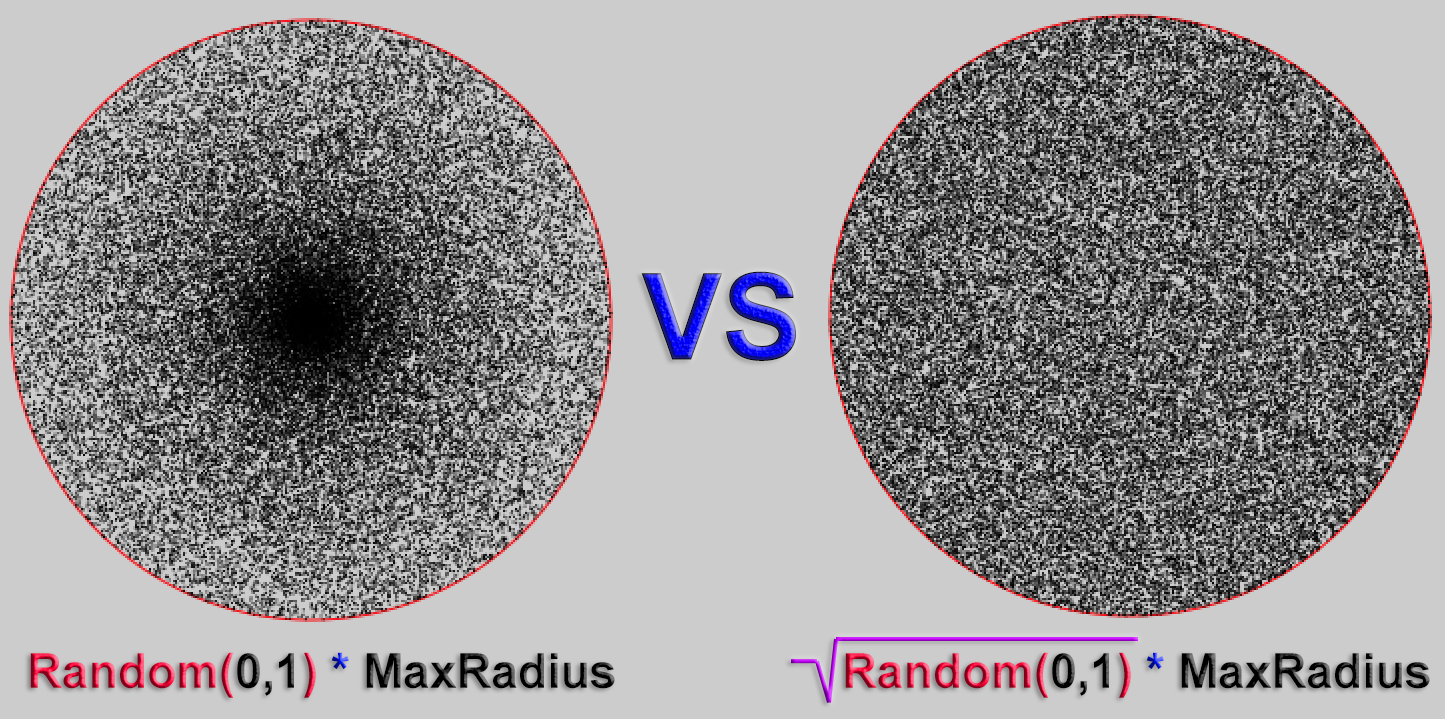Figure 8 was generated via the following Processing sketch.

###### Code Sample:

If Unity is your game engine of choice, you can pick a random uniform point like so,

### Conclusion

Hopefully by now you have gained insight with regards to picking a uniformly distributed point in a circle.
Using polar coordinates gets you mostly there but you must also take the circle’s area into account. We do that by using the area of a circle formula to make our random radius proportional to the area of the circle. Most popular game engines like Unity already support a method to pick random points in a circle. However you should now understand what is happening under the hood. Until next time!

Share It!

## One thought on “Random Points In A Circle”

1. erichlof says:

Hi Brandon,
Thank you for another well-written, clearly-explained article. I have seen this formula tossed around in source code, (and even in shader code on ShaderToy.com and glslSandbox.com) but I didn’t really know why that sqrt() was in there. Now it makes sense!
Thanks again,
-Erich

This site uses Akismet to reduce spam. Learn how your comment data is processed.